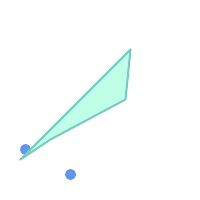## Name

ST_MinkowskiSum — Performs Minkowski sum

## Synopsis

`geometry ST_MinkowskiSum(`geometry geom1, geometry geom2`)`;

## Description

This function performs a 2D minkowski sum of a point, line or polygon with a polygon.

A minkowski sum of two geometries A and B is the set of all points that are the sum of any point in A and B. Minkowski sums are often used in motion planning and computer-aided design. More details on Wikipedia Minkowski addition.

The first parameter can be any 2D geometry (point, linestring, polygon). If a 3D geometry is passed, it will be converted to 2D by forcing Z to 0, leading to possible cases of invalidity. The second parameter must be a 2D polygon.

Implementation utilizes CGAL 2D Minkowskisum.

Availability: 2.1.0This method needs SFCGAL backend.

## Examples

Minkowski Sum of Linestring and circle polygon where Linestring cuts thru the circleBefore SummingAfter summing
```SELECT ST_MinkowskiSum(line, circle))
FROM (SELECT
ST_MakeLine(ST_MakePoint(10, 10),ST_MakePoint(100, 100)) As line,
ST_Buffer(ST_GeomFromText('POINT(50 50)'), 30) As circle) As foo;

-- wkt --
MULTIPOLYGON(((30 59.9999999999999,30.5764415879031 54.1472903395161,32.2836140246614 48.5194970290472,35.0559116309237 43.3328930094119,38.7867965644036 38.7867965644035,43.332893009412 35.0559116309236,48.5194970290474 32.2836140246614,54.1472903395162 30.5764415879031,60.0000000000001 30,65.8527096604839 30.5764415879031,71.4805029709527 32.2836140246614,76.6671069905881 35.0559116309237,81.2132034355964 38.7867965644036,171.213203435596 128.786796564404,174.944088369076 133.332893009412,177.716385975339 138.519497029047,179.423558412097 144.147290339516,180 150,179.423558412097 155.852709660484,177.716385975339 161.480502970953,174.944088369076 166.667106990588,171.213203435596 171.213203435596,166.667106990588 174.944088369076,
161.480502970953 177.716385975339,155.852709660484 179.423558412097,150 180,144.147290339516 179.423558412097,138.519497029047 177.716385975339,133.332893009412 174.944088369076,128.786796564403 171.213203435596,38.7867965644035 81.2132034355963,35.0559116309236 76.667106990588,32.2836140246614 71.4805029709526,30.5764415879031 65.8527096604838,30 59.9999999999999)))
```

Minkowski Sum of a polygon and multipointBefore SummingAfter summing: polygon is duplicated and translated to position of points
```SELECT ST_MinkowskiSum(mp, poly)
FROM (SELECT 'MULTIPOINT(25 50,70 25)'::geometry As mp,
'POLYGON((130 150, 20 40, 50 60, 125 100, 130 150))'::geometry As poly
) As foo

-- wkt --
MULTIPOLYGON(
((70 115,100 135,175 175,225 225,70 115)),
((120 65,150 85,225 125,275 175,120 65))
)
```